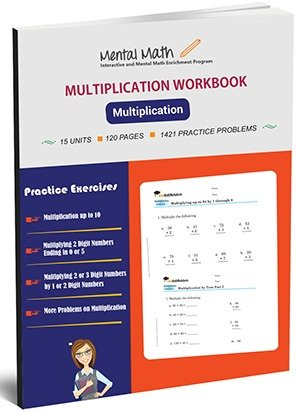20,864

# Multiplication Worksheets\$7.00

In our math multiplication worksheets, students are introduced to the concepts of multiplication as a repeated addition. The separate multiplication table of 2 to 10 in different exercises will describe how multiplication is related with the addition. Same as addition and subtraction, students begin multiplication exercise, multiplying 2 to all numbers up to 10. Similarly, they will continue for numbers 5, 10, 3, 4, and onward. Each exercise in multiplication will increase in variety and complexity to make students efficient in multiplication concepts. Multiplying 2 or 3 digit numbers by 1 or 2 digit numbers and multiplication by tens, by hundreds, and by thousands are the advanced problems in 15 units long multiplication section.

Worksheet # Topics/Curriculum # of Pages Sample Add to cart
Unit-1 Multiplication by 2, by 5, and by 10 8 pages\$1.00

Unit-2 Multiplication by 3, by 4, and by 6 8 pages\$1.00

Unit-3 Multiplication by 7, by 8, and by 9 8 pages\$1.00

Unit-4 Multiplication up to 10 8 pages\$1.00

Unit-5 Multiplying 2 Digit Numbers by 2, by 3, and by 4 8 pages\$1.00

Unit-6 Multiplying 2 Digit Numbers by 2 through 7 8 pages\$1.00

Unit-7 Multiplying 2 Digit Numbers by 2 through 9 8 pages\$1.00

Unit-8 Multiplying 2 Digit Numbers Ending in 0 or 5 8 pages\$1.00

Unit-9 Multiplying 2 Digit Numbers by 1 Digit Numbers 8 pages\$1.00

Unit-10 Multiplying 3 or More Digit Numbers by 1 Digit Numbers 8 pages\$1.00

Unit-11 Multiplying 2-Digit Numbers by 2-Digit Numbers Part I 8 pages\$1.00

Unit-12 Multiplying 2-Digit Numbers by 2-Digit Numbers Part II 8 pages\$1.00

Unit-13 Multiplying 2 or 3 Digit Numbers by 2-Digit Numbers 8 pages\$1.00

Unit-14 Multiplying 2 or 3 Digit Numbers by 1 or 2 Digit Numbers 8 pages\$1.00

Unit-15 More Problems on Multiplication 8 pages\$1.00

## Choose From Over 15 Worksheets That Best Suit Your Child’s Skill Level and Unique Needs

You can purchase our high quality math worksheets separately, or purchase an entire book and save 40%!

### Equation Worksheets

Evaluate Algebraic Expressions and Solve Equations

See all units

### Integers Worksheets

Four Operations on integers and their Simplification

See all units

### Decimals Worksheets

Decimal Concept and Four Operations on Decimals

See all units

### Fractions Worksheets

Fraction Concepts and Four Operations on Fractions

See all units

### Division Worksheets

Dividing up to 4 or more digit Numbers by 2 or 3-digit Numbers

See all units

### Multiplication Worksheets

Multiplication Table of 2 through 10 and Multiplication by Thousands

See all units

### Subtraction Worksheets

Subtracting 4 to 1-digit Numbers form 4 digit Numbers

See all units